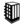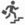Write a Program to find two maximum numbers in an array# Search Interview QuestionsMore than 3000 questions in repository.There are more than 900 unanswered questions.Have a video suggestion.
Click Correct / Improve and please let us know.
Label / Company      Label / Company / TextSubmit Question

Interview Questions and AnswersQ1. Can we reduce the visibility of the overridden method ? Core Java
Ans. NoHelp us improve. Please let us know the company, where you were asked this question :LikeDiscussCorrect / Improveoverriding   access specifier   oops   java   yes-noQ2. Write a Program to swap 2 variables ( using 3rd variable ) Core Java
Ans. int x = 1;
int y = 2;
int z = x;
x = y;
y = z;

System.out.println("x="+x);
System.out.println("y="+y);Help us improve. Please let us know the company, where you were asked this question :LikeDiscussCorrect / Improveswap 2 variables  code  codingAsked in 1 CompaniesbasicQ3. Write a Program to swap two variables ( without using 3rd variable )
Ans.
int x = 1;
int y = 2;

x = x+y;
y = x-y;
x = x-y;

System.out.println("x="+x);
System.out.println("y="+y);Help us improve. Please let us know the company, where you were asked this question :LikeDiscussCorrect / Improveswap 2 variablesQ4. Write a Program to find two maximum numbers in an array
Ans. public class FindTwoMax {
public static void main(String[] args) {
int myArray[] = { 1, 3, 5, 8, 6, 3 };
int max1 = myArray;
int max2 = 0;
for (int count = 0; count < myArray.length; count++) {
if (max2 < myArray[count]) {
max2 = max1;
max1 = myArray[count];
} else if (max2 < myArray[count]) {
max2 = myArray[count];
}
}
System.out.println(max1);
System.out.println(max2);
}
}Help us improve. Please let us know the company, where you were asked this question :LikeDiscussCorrect / Improvefind 2 max in array

## Help us and Others Improve. Please let us know the questions asked in any of your previous interview.

Any input from you will be highly appreciated and It will unlock the application for 10 more requests.

 Company Name: Questions Asked: